﻿ DFT hampers atomic force microspopy

# Useless  quantum method

## Now, we can manipulate a single atom !

### [ But quantum mechanics stops us from moving forward. ]

(Fig.1)  Atomic force microscope can detect and manipulate an atom.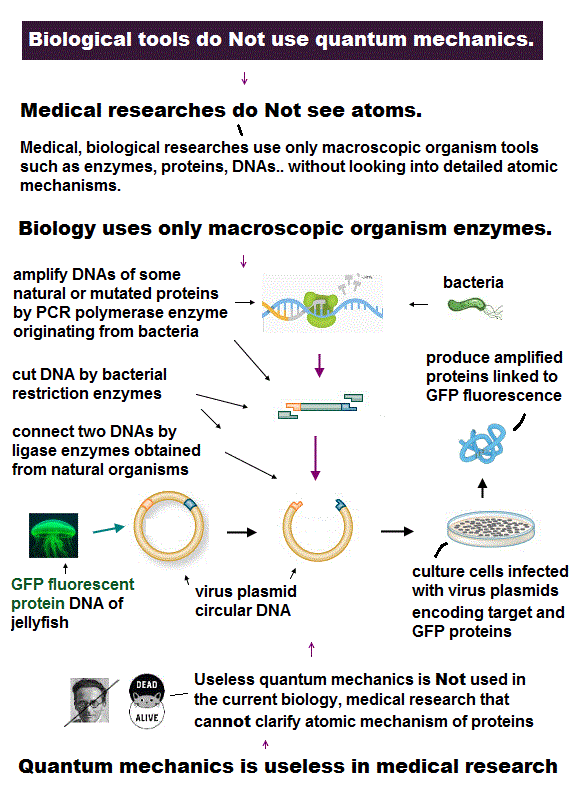Now, using atomic force microscope we can detect slight atomic force and even manipulate each single atom.

Utilizing these atomic force, we could realize "atomic tweezers" which can pick up a single atom to build nano-machine ?

But useless quantum mechanics stops us from moving forward to construct atomic-level nano-machine !

## No real electrons in quantum mechanics !

### [ Quantum wavefunction cannot express clear electron's motion. ]

(Fig.2)  After measuring force → useless quantum mechanical model.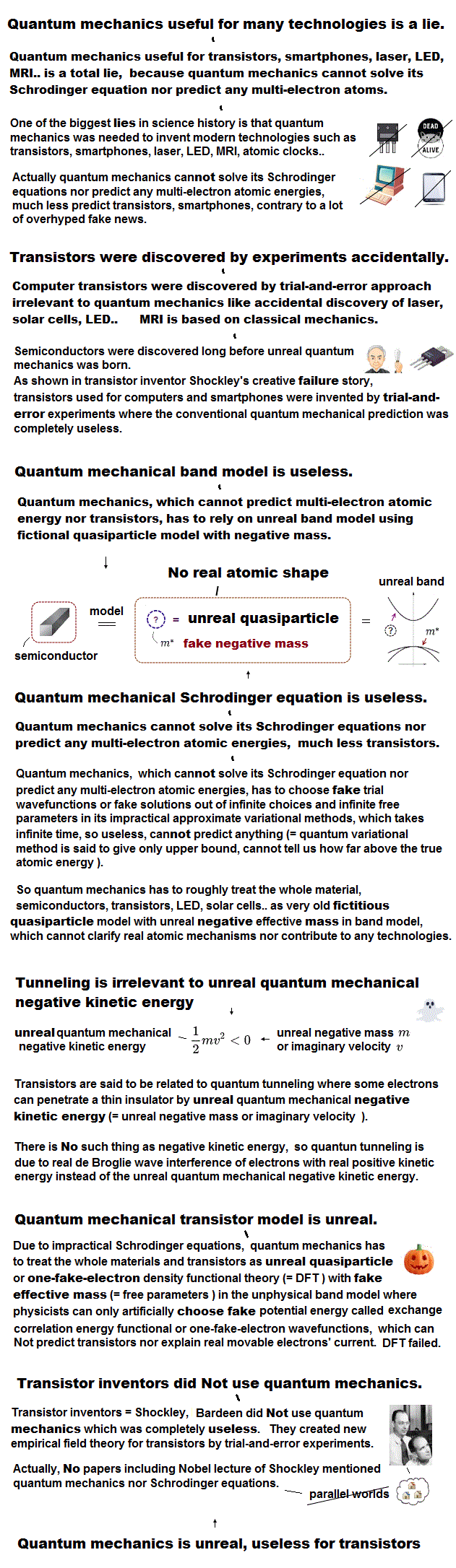Now we have the technology of measuring and manipulating each single atom.  The problem is we do NOT have a proper model to express these atomic behavior !

After measuring each atomic force, there is the only useless Schrödinger equation as a calculating tool in quantum mechanics.

Quantum wave function has NO realistic concepts such as "moving electrons", because it cannot specify the positions and velocity of each electron.

Unless we can describe each "real" electron, it's impossible to predict dynamic atomic behavior under some external electric field or chemical reactions !

## Useless quantum mechanics is the only option !

### [ Quantum mechanics has NO solution in multi-electron atoms. ]

(Fig.3)  They just choose "fake solution" from infinite choices ← useless !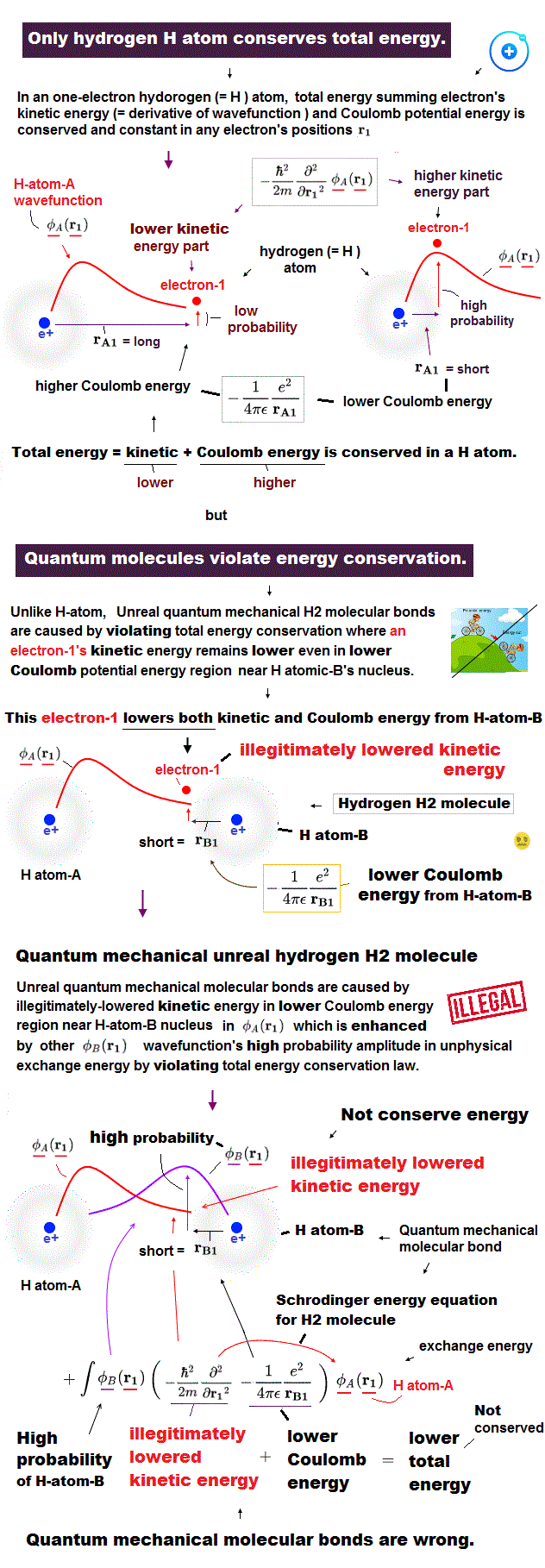After detecting the slight force of a single atoms or molecule, we need to interpret those forces using some atomic model.

Unless we can explain each single atomic force, we cannot move forward to the next step of utilizing multi-atomic bahavior.

The problem is that there is the only useless quantum mechnaics (= Schrödinger equation ) as a tool to interpret these atomic data, now.

Quantum mechanics have NO exact solution in multi-electron atoms, so they just choose fake solution from infinite choices, meaning no power to predict anyhing.

In atomic force microspopy, they use densisty functional theory (= DFT ), which is a further approximation of quantum mechanics, so more incorrect and useless.

## Density functional theory (= DFT ) is useless.

### [ DFT just "chooses" fake potential from infinite choices, so useless. ]

(Fig.4)  DFT is one of "false" approximations of Schrödinger equation.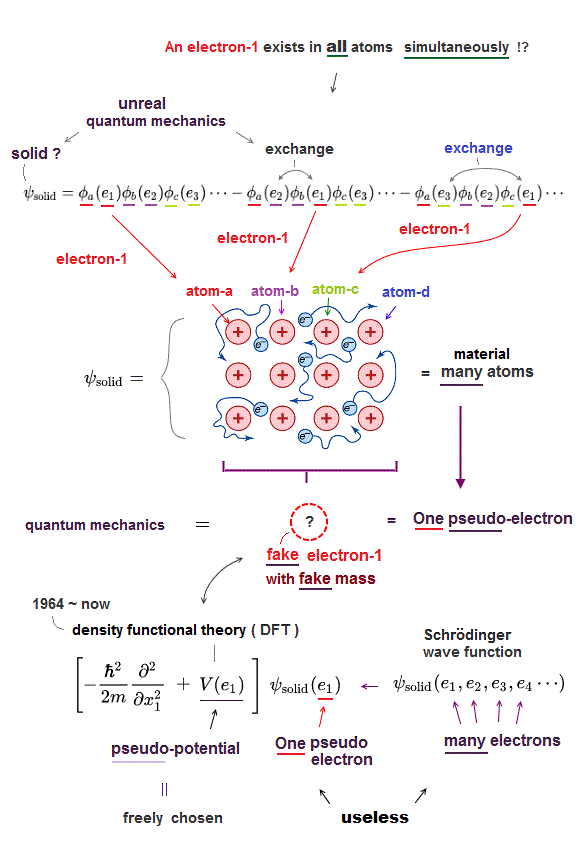In atomic force microcopy, the only tool is useless density functional theory (= DFT ).  All papers rely on this DFT ( this , this ).

Density functional theory (= DFT ) is one of approximate Schrödinger equations.  DFT replaces electron-electron interaction by fake artificial potential.

In Schrödinger equation, they just choose fake approximate solutions as "trial wavefunction".  In DFT, they have to choose "fake artificial potential", too.

There are NO restrictions in choosing these fake potentials.  We can choose any forms of these potentials from infinite choices in DFT.

So DFT has NO ability to predict any atomic behavior.  In spite of this, this DFT is the only tool for analysing atomic force microcsopy !

No matter how many times they catch slight atomic force, all they can use is useless density functional theory (= DFT, see this p.6 ).

All in these single molecule detections, useless density functional theory is the only method to interpret each atomic force ( see abstract of this and this ).

This is where out science stops.  Physicists are shackled to old useless methods which don't admit "concrete force".  It just gives us weird wavefunctions as a tool.

## Choosing "fake potential" in DFT.

### [ DFT chooses fake potential, solution from infinite choices. ]

(Fig.5)  So DFT cannot predict any atomic behavior, useless.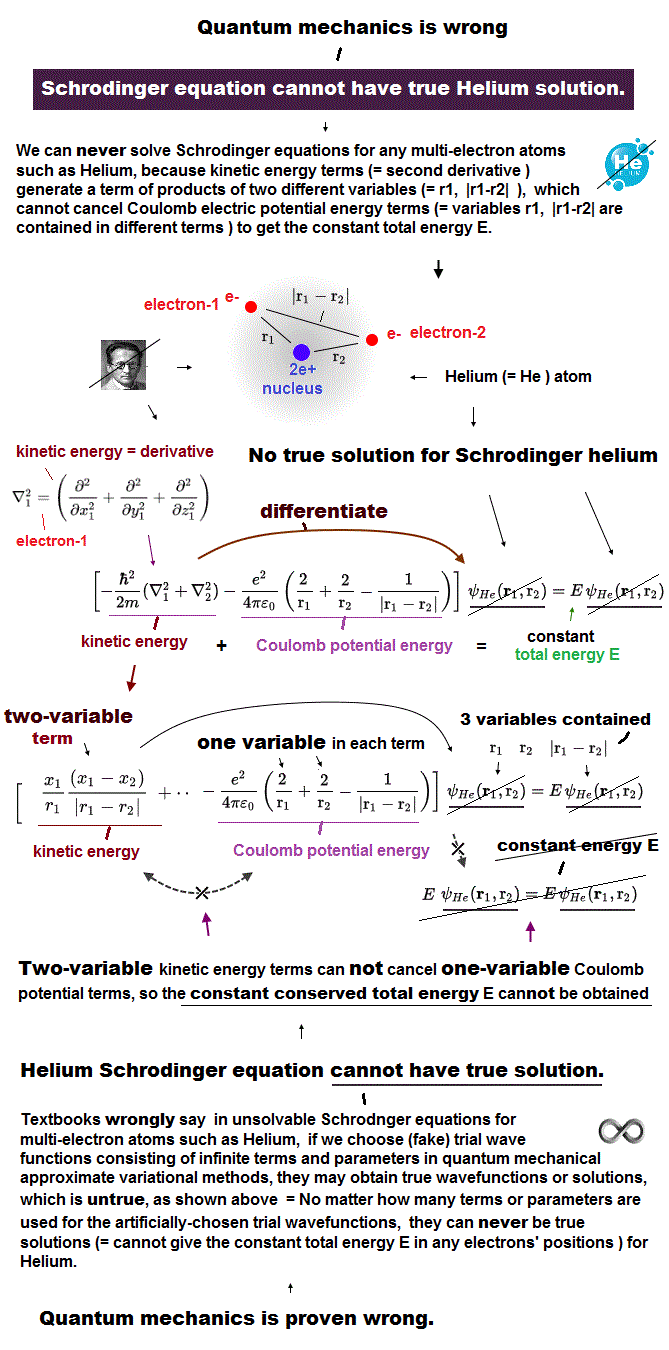Density functional theory (= DFT ) forcedly changes muti-electron equation into one-electron approximation.

In one-electron DFT method, we need to artificially pick up "fake" forms of electrons' interaction potential called exchange correlation functional.

Selecting convenient functional for your purpose is all you have to do in DFT ( this p.9 ).  There is NO universal functional describing any potential ( this p.2 ).

When you pick up one of functional ( ex. LDA ) from infinite choices and it fails, you can pick up other functionals as fake electrons' potential.

Selecting fake potential and fake solutions as you like means this DFT method has NO ability to predict any atomic behavior, so useless, too

## "Real" atomic model is necessary.

### [  ① Introduce real atomic model → ② formulate atomic force  ]

(Fig.6)  Introducing real electrons' motion, real de Broglie wave is essential.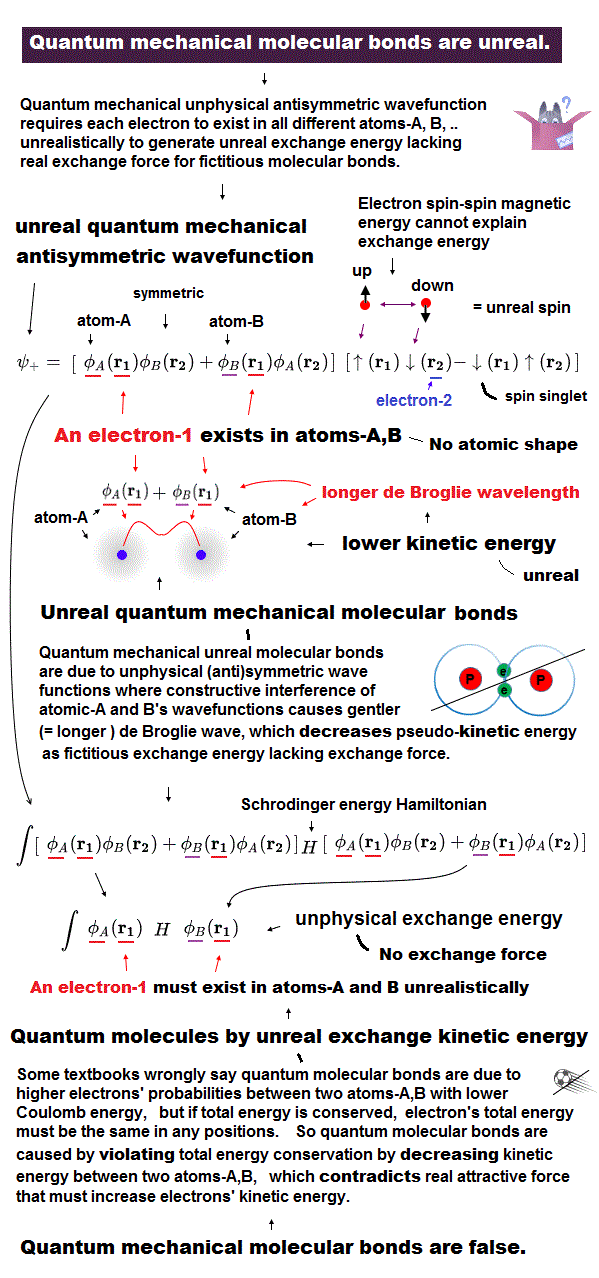We can measure each atomic force using atomic force microscope, but quantum mechanics cannot formulate these forces using 'concrete' models !

The first thing to do is introduce real and simple atomic models.  Quantum useless wave function cannot separate each single electron.

So in Schrödinger equation, two electrons cannot avoid each other by Coulomb repulsions in the simple way, which makes it very hard to formulate atomic force.

Not only Coulomb forces but also de Broglie wave is necessary to estimate each atomic radius ( atomic radius is longer as 1, 2, 3 × .. de Broglie wavelength ).

Interference among electrons' de Broglie waves can explain well about Pauli exclusion force and valence electron number.

Quantum Schrödinger equation does NOT admit real de Broglie wave, so it cannot estimate actual atomic radius.

So under vague quantum wave function (= fake solution in multi-electrons ), it's impossible to formulate actual interatomic force and atomic tweezers.2017/3/24 updated. Feel free to link to this site.